## ISP图像信号处理——白平衡校正和标定介绍以及C++实现

news/2023/12/2 17:29:33/

http://t.csdn.cn/HuI67

## 白平衡校正

• 手动白平衡：在拍照前通过拍摄一个18度灰的卡片，然后计算出当时环境的白平衡增益值对后面的图片进行校正；

• 自动白平衡：相机通过本身的算法，通过获取的图像自动计算出增益值对图像进行校正的方式。

### AWB(Automatic White Balance)，自动白平衡理解

1.一部分厂家将（R/G, B/G）定义为sensor捕捉到的图像中红色和蓝色的统计值，因此在高色温（如D65）下B/G大于R/G。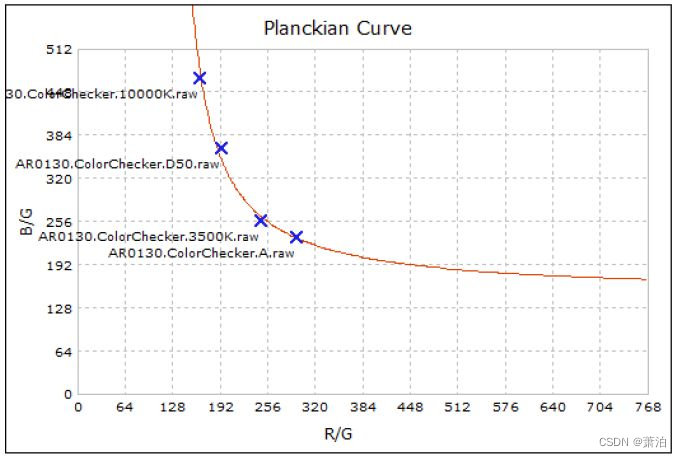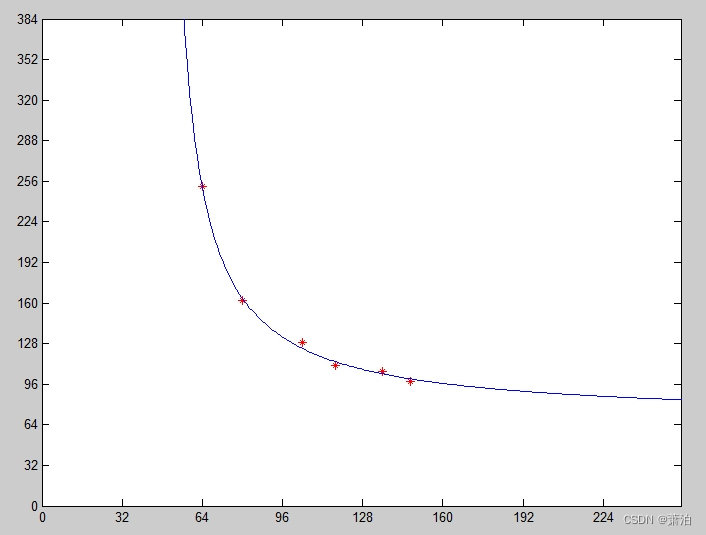2.另一部分厂家则将（R/G, B/G）定义成为了使sensor捕捉的图像达到白平衡需要施加的增益系数，因此在高色温下B/G小于R/G。

• 以标定好的若干个色温点（A, TL84, D50, D65, D75等）为核心可以拟合出一条色温曲线
• 以色温曲线上的点为圆心，以一定半径画椭圆，可以得到一些列椭圆。
• 所有椭圆所覆盖的（R/G，B/G）集合就认为是真实场景中的白色物体在全部色温下的可能值。不落在这个范围内的颜色不认为是白色。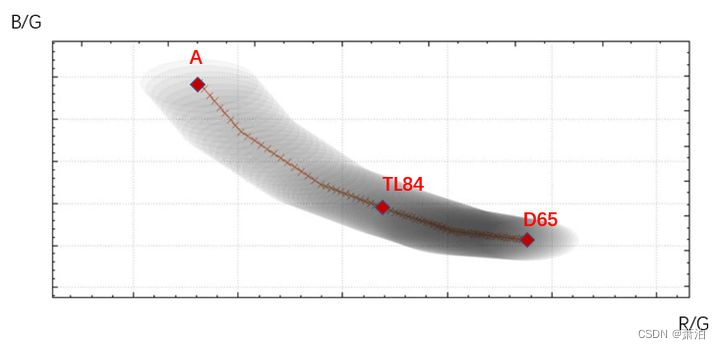1. 在第N帧图像结束时ISP硬件采集关于白平衡的统计信息
2. 白平衡算法根据第N帧的统计数据预测第N+1帧的控制参数
3. 摄像机固件在第N+1帧图像到达之前将白平衡控制参数写入ISP硬件寄存器
4. 第N+1帧图像使用新的白平衡参数进行曝光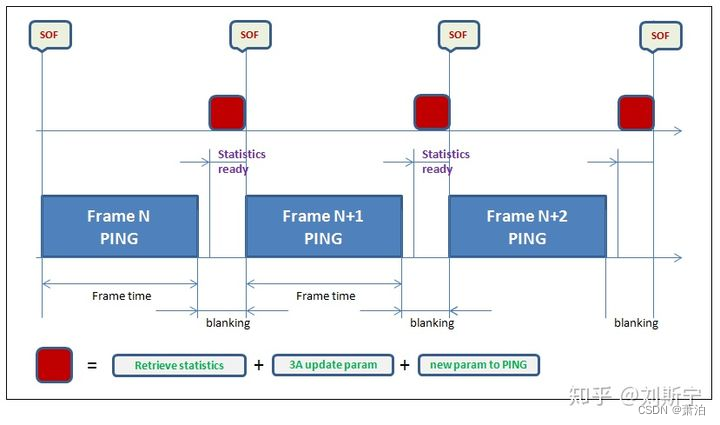1. 在第N帧图像结束时ISP硬件采集关于白平衡的统计信息
2. 在第N+1帧开始后，白平衡算法根据第N帧的统计数据预测第N+2帧的控制参数，需要在第N+1帧结束前完成所有计算
3. 摄像机固件在第N+2帧图像到达之前将新的白平衡控制参数写入ISP硬件寄存器
4. 第N+2帧图像使用新的白平衡参数进行曝光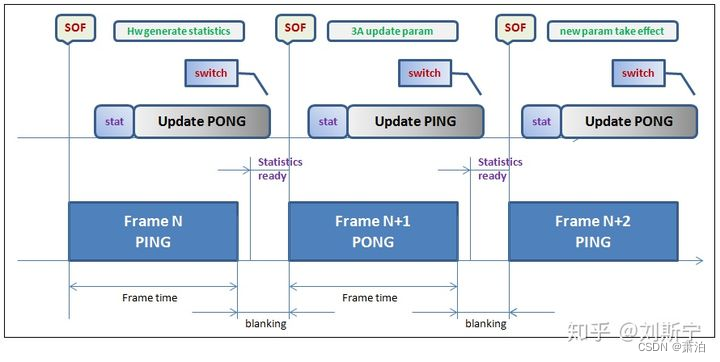### 白平衡校正算法

#### 1.灰度世界算法

• 计算各个颜色通道的平均值；
• 寻找一个参考值K，一般情况选取Gmean;
• 计算Rgain = Gmean/Rmean, Bgain = Gmean/Bmean;
• 对图像中的每个像素都乘以对应的gain值进行校正；
• 以下C++代码，不知道本人缺少什么运行条件，在自己电脑上最后显示结果不太理想；大家也可以试试，该代码出自：http://t.csdn.cn/edydD
``````//自动白平衡  //灰度世界算法
void GrayWorldAlgorithm(Mat& src,Mat& dst)
{assert(3==src.channels());//求BGR分量均值auto mean = mean(src);//需要调整的BGR分量的增益float gain_B(0),gain_G(0),gain_R(0);float K = (mean+mean+mean)/3.0f;gain_B = K/mean;gain_G = K/mean;gain_R = K/mean;vector<Mat> channels;split(src,channels);//调整三个通道各自的值channels = channels*gain_B;channels = channels*gain_G;channels = channels*gain_R;//通道合并cv::merge(channels,dst);
}``````

``````//灰度世界法，输入三通道彩色图
Mat awbgray(Mat img_rgb8)
{Mat img_awb = Mat::zeros(height, width, CV_32FC3);Mat imgR = Mat::zeros(height, width, CV_8UC1);Mat imgG = Mat::zeros(height, width, CV_8UC1);Mat imgB = Mat::zeros(height, width, CV_8UC1);double Rsum=0, Gsum=0,Bsum=0;/*unsigned char* srcdata;unsigned char *dstdata;*/for (int row = 0; row < height; row++){//ptr得到行数据的头指针，得到row行指针/*uchar* data = img_awb.ptr<uchar>(row);*/Vec3b* inptr = img_rgb8.ptr<Vec3b>(row);for (int col = 0; col < width; col++){//BGGR分布？//imgB.at<Vec3b>(row, col)   b通道/*uchar bdata = data[col * img_awb.channels() + 0];*/imgB.at<uchar>(row, col) = (*(inptr + col));imgG.at<uchar>(row, col) = (*(inptr + col));imgR.at<uchar>(row, col) = (*(inptr + col));Bsum += imgB.at<uchar>(row, col);Gsum += imgG.at<uchar>(row, col);Rsum += imgR.at<uchar>(row, col);}}double Rmean=0, Gmean=0, Bmean = 0;Rmean = Rsum / (height * width);Gmean = Gsum / (height * width);Bmean = Bsum / (height * width);double K = (Rmean + Gmean + Bmean) / 3;cout << "Rmeanvalue:" << Rmean << endl;cout << "Gmeanvalue:" << Gmean << endl;cout << "Bmeanvalue:" << Bmean << endl;cout << "rgbmeanvalue:" << K << endl;double Rgain = K / Rmean;double Ggain = K / Gmean;double Bgain = K / Bmean;//重新调整计算RGB值for (int row = 0; row < height; row++){Vec3b* inptr = img_rgb8.ptr<Vec3b>(row);Vec3f* outptr = img_awb.ptr<Vec3f>(row);for (int col = 0; col < width; col++){(*(outptr + col)) = Rgain * (*(inptr + col));(*(outptr + col)) = Ggain * (*(inptr + col));(*(outptr + col)) = Bgain * (*(inptr + col));}}//convertScaleAbs(img_awb, img_awb);//转为CV_8UC1/*imshow("img_awb", img_awb);waitKey(0);*/return img_awb;
}``````

（左为输入，右为输出，不是一一对应的截图）：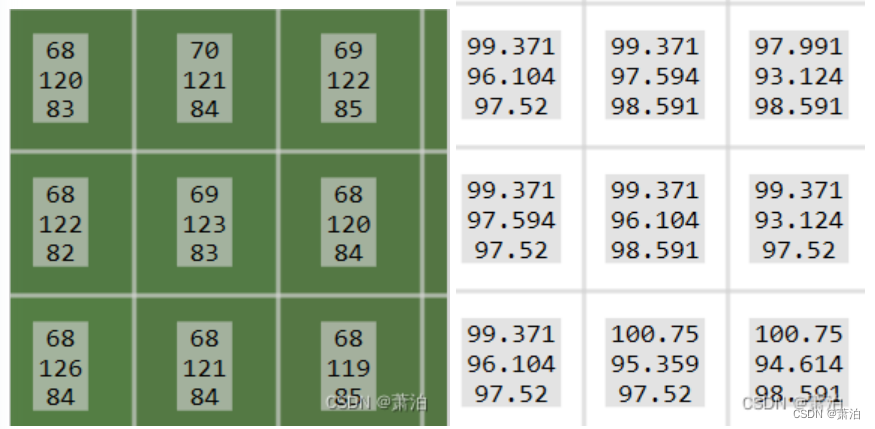#### 2.完美反射法

(perfect Reflector)基于这样一种假设，一幅图像中最亮的像素相当于物体有光泽或镜面上的点，它传达了很多关于场景照明条件的信息。如果景物中有纯白的部分，那么就可以直接从这些像素中提取出光源信息。因为镜面或有光泽的平面本身不吸收光线，所以其反射的颜色即为光源的真实颜色，这是因为镜面或有光泽的平面的反射比函数在很长的一段波长范围内是保持不变的。那么在这个假设下，图像中就一定存在一个纯白色的的像素或者最亮的点。

（1）遍历原始图像，统计RGB三通道之和的直方图；
（2）遍历原始图像，找到RGB三通道各自的最大值Bmax、Gmax、Rmax
（3）设定比例 r ，对RGB之和的直方图进行倒叙遍历，找到使白点像素个数超过总像素个数比例的阈值，T；
（4）遍历原始图像，计算RGB之和大于 T 的像素，各个通道取平均，得到Bavg、Gavg、Ravg；
（5）遍历原始图像，分别计算RGB三通道的调整值Aout=A / Aavg * Amax；
（6）防溢出处理，这里可以采用简单的截断即可。

``````​
//白平衡校正,完美反射法,使用比例r和阈值T，
Mat awbreflect2(Mat img_rgb8)
{int histrgbsum[255 * 3 + 1] = { 0 };double Rmax = 0, Gmax = 0, Bmax = 0;//uchar maxrgb = { 0 };for (int row = 0; row < height; row++){const uchar* inptr = img_rgb8.ptr<uchar>(row);for (int col = 0; col < width; col++){//统计RGB三通道之和的直方图int sum = *(inptr + 3 * col) + *(inptr + 3 * col + 1) + *(inptr + 3 * col + 2);histrgbsum[sum]++;//找到RGB三通道各自的最大值Bmax、Gmax、RmaxBmax = max(Bmax, (double)*(inptr + 3 * col));Gmax = max(Gmax, (double)*(inptr + 3 * col + 1));Rmax = max(Rmax, (double)*(inptr + 3 * col + 2));/*maxrgb = max(maxrgb, *(inptr + 3 * col));maxrgb = max(maxrgb, *(inptr + 3 * col+1));maxrgb = max(maxrgb, *(inptr + 3 * col+2));*/}}//设定比例r为10%double num = 0,ratio=0.1;int threshold = 0;int len = 0;len=sizeof(histrgbsum) / sizeof(histrgbsum);//int len = end(histrgbsum) - begin(histrgbsum);cout << "histagram length:" << len << endl;for (len; len >= 0; len--){num += histrgbsum[len];//计算R+G+B的数量超过像素总数的ratio的像素值if (num > height * width * ratio){//使白点像素个数超过总像素个数的比例时，为阈值Tthreshold = len;break;}}//计算RGB之和大于 T 的像素，对大于阈值的像素各通道取平均，得到Bavg、Gavg、Ravg；double Rsum = 0, Gsum = 0, Bsum = 0;double Ravg = 0, Gavg = 0, Bavg = 0;int pixnum = 0;for (int row = 0; row < height; row++){const uchar* inptr = img_rgb8.ptr<uchar>(row);for (int col = 0; col < width; col++){//计算RGB之和，上面的局部变量又用一遍int sum = *(inptr + 3 * col) + *(inptr + 3 * col + 1) + *(inptr + 3 * col + 2);if (sum > threshold){Bsum += *(inptr + 3 * col);Gsum += *(inptr + 3 * col + 1);Rsum += *(inptr + 3 * col + 2);pixnum++;}}}Ravg = Rsum / (double)pixnum;Gavg = Gsum / (double)pixnum;Bavg = Bsum / (double)pixnum;//创建与输入图像一样大小类型的矩阵Mat img_awb = Mat::zeros(img_rgb8.size(), img_rgb8.type());//量化0-255,重新计算RGB值,分别计算RGB三通道的调整值Aout=A / Aavg * Amaxdouble Rout = 0, Gout = 0, Bout = 0;for (int row = 0; row < height; row++){const uchar* inptr = img_rgb8.ptr<uchar>(row);uchar* outptr = img_awb.ptr<uchar>(row);for (int col = 0; col < width; col++){Bout = (double)*(inptr + 3 * col) / Bavg * Bmax;Gout = (double)*(inptr + 3 * col+1) / Gavg * Gmax;Rout = (double)*(inptr + 3 * col+2) / Ravg * Rmax;Bout = min(max((double)0, Bout), (double)255);Gout = min(max((double)0, Gout), (double)255);Rout = min(max((double)0, Rout), (double)255);//将计算好的RGB值赋给新矩阵*(outptr + 3 * col) = (uchar)Bout;*(outptr + 3 * col+1) = (uchar)Gout;*(outptr + 3 * col+2) = (uchar)Rout;}}return img_awb;}​``````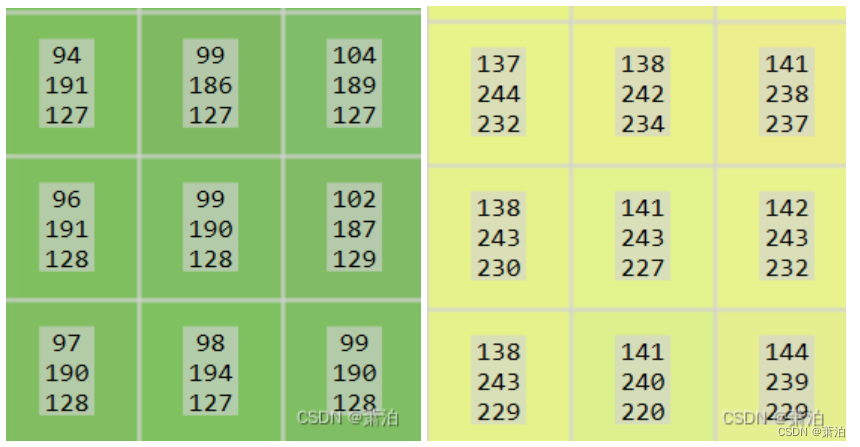#### 3.动态阈值法

YUV颜色空间（亦称YCrCb）主要用于优化彩色视频信号的传输，Y表示亮度，U和V表示色度（色调和饱和度）。亮度是通过RGB输入信号来建立的，方法是将RGB信号的特定部分叠加到一起。“色度”则定义了颜色的两个方面─色调与饱和度，分别用Cr和Cb来表示。其中，Cr反映了RGB输入信号红色部分与RGB信号亮度值之间的差异。而Cb反映的是RGB输入信号蓝色部分与RGB信号亮度值之同的差异。

(1)把图像w*h从RGB空间转换到YCrCb空间。转换公式如下：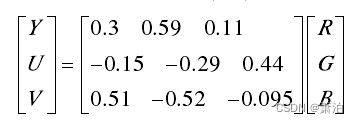(2)通过限定YUV的区域来判断是否为白点，通过四个限制条件俩限制白点，满足条件的点就是白点，参与后续的计算，否则，点直接舍弃。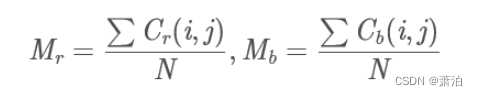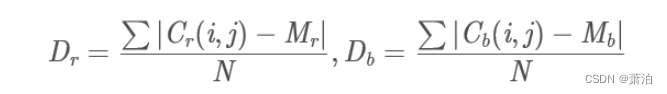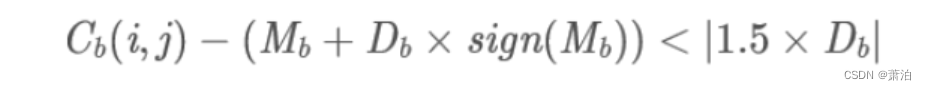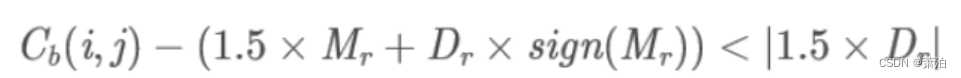(1)选取参考“参考白色点”中最大的10%的亮度（Y分量）值，并选取其中的最小值Lu_min;

(2)调整RL，若RL(i,j)<Lu_min, RL(i,j)=0; 否则，RL(i,j)=1；

(3)分别把R，G，B与RL相乘，得到R2，G2，B2。 分别计算R2，G2，B2的平均值，得到Rav，Gav，Bav；

(4)得到调整增益： Ymax=double(max(max(Y));

Rgain=Ymax/Rav;

Ggain=Ymax/Gav;

Bgain=Ymax/Bav;

(5)调整原图像：Ro= R*Rgain; Go= G*Ggain; Bo= B*Bgain。

OpenCV图像处理专栏十一 | IEEE Xplore 2015的图像白平衡处理之动态阈值法 (qq.com)

### 【多任务案例：猫狗脸部定位与分类】

【猫狗脸部定位与识别】 1 引言2 损失函数3 The Oxford-IIIT Pet Dataset数据集4 数据预处理4 创建模型输入5 自定义数据集加载方式6 显示一批次数据7 创建定位模型8 模型训练9 绘制损失曲线10 模型保存与预测 1 引言 猫狗脸部定位与识别分为定位和识别&#xff0c;即定位猫狗…

### 动态链接那些事

1、为什么要动态链接 1.1 空间浪费 对于静态链接来说&#xff0c;在程序运行之前&#xff0c;会将程序所需的所有模块编译、链接成一个可执行文件。这种情况下&#xff0c;如果 Program1 和 Program2 都需要用到 Lib.o 模块&#xff0c;那么&#xff0c;内存中和磁盘中实际上就…

### 已解决 Bug——IndexError: index 3 is out of bounds for axis 0 with size 3问题

&#x1f337;&#x1f341; 博主猫头虎&#xff08;&#x1f405;&#x1f43e;&#xff09;带您 Go to New World✨&#x1f341; &#x1f984; 博客首页: &#x1f405;&#x1f43e;猫头虎的博客&#x1f390;《面试题大全专栏》 &#x1f995; 文章图文并茂&#x1f996…

### 十四天学会C++之第三天（数组和字符串）

1. 数组的定义和初始化 数组是一种由相同数据类型的元素组成的集合&#xff0c;这些元素按照一定的顺序存储在连续的内存位置上。数组的大小在创建时是固定的&#xff0c;无法在运行时改变。 在C中&#xff0c;数组的定义和声明非常简单。定义一个数组&#xff1a; 数据类型…

### YTM32的电源管理与低功耗系统详解

YTM32的电源管理与低功耗系统详解 苏勇&#xff0c;2023年10月 文章目录 YTM32的电源管理与低功耗系统详解缘起原理与机制电源管理模型的功耗模式正常模式&#xff08;Normal&#xff09;休眠模式&#xff08;Sleep&#xff09;深度休眠模式&#xff08;DeepSleep&#xff09;…